# Base change

change of base

A category-theoretical construction; special cases are the concept of an induced fibration in topology, and extension of the ring of scalars in the theory of modules.

Let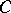be a category with fibred products and letbe a morphism of. A base change by means ofis a functor from the category of-objects (i.e. the category of morphism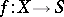, whereis an object of) to the category of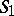-objects, taking an-objectto the-object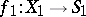, where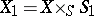and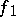is projection onto the second factor. The morphismis then called the base-change morphism. One also says that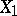is obtained fromby base change.

A special case of a base change is the concept of a fibre of a morphismof schemes: The fibre of the morphismover a point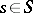is the scheme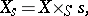i.e. the scheme obtained fromby base change via the natural morphism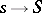. A similar definition yields the geometric fibre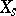; it is obtained by base change via the morphism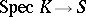associated with a geometric point of, whereis an algebraically closed field. Many properties of the-schemeare preserved under a base change. The inverse problem — to infer the properties of a schemefrom those of the schemes obtained fromby base change — is considered in descent theory (see also ).

Letbe the morphism obtained fromvia a morphism, so that one has a Cartesian square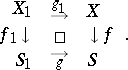Let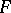be a sheaf of sets on. Then there exists a natural sheaf mapping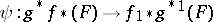. Ifis a sheaf of Abelian groups, then for everythere exists a natural sheaf homomorphism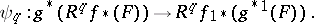Under these conditions,andare also called base-change morphisms. It is usually said that the base-change theorem is valid if(or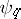) is an isomorphism. In other words, the base-change theorem is a proposition about the compatibility (commutability) of the functorswith the base-change functor. In particular, if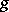is the imbedding of a point, the base-change theorem states that there exists a natural isomorphismbetween the fibre of the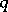-th direct image of the sheafand the-dimensional cohomology group of the fibre of the morphism. The base-change theorem is valid in the following situations: 1)is a proper mapping of paracompact topological spaces,is a locally compact space ; 2)is a separable quasi-compact morphism of schemes,is a flat morphism,is a quasi-coherent sheaf of-modules (the comparison theorem for the cohomology of ordinary and formal schemes — see  — can also be interpreted as a base-change theorem); or 3)is a proper morphism of schemes,is a torsion sheaf in the étale topology. Some other cases in which base-change theorems are valid are considered in .